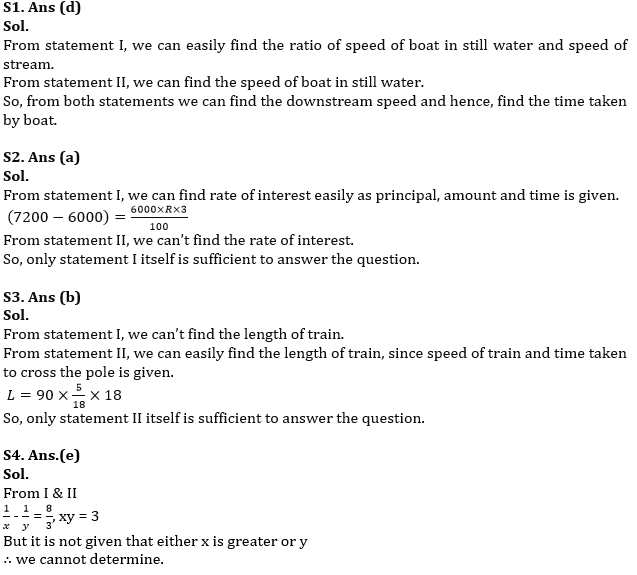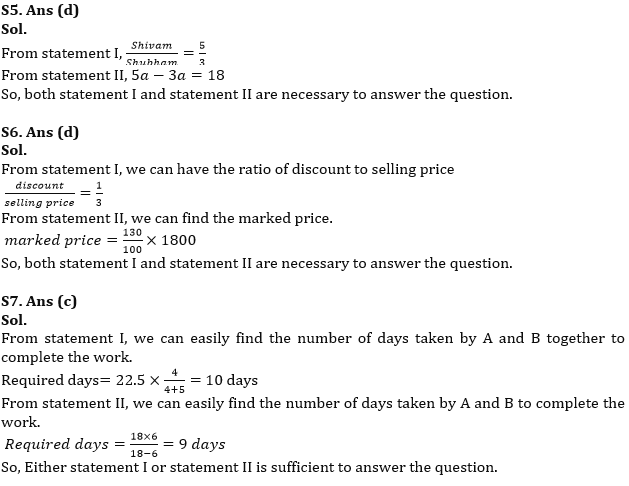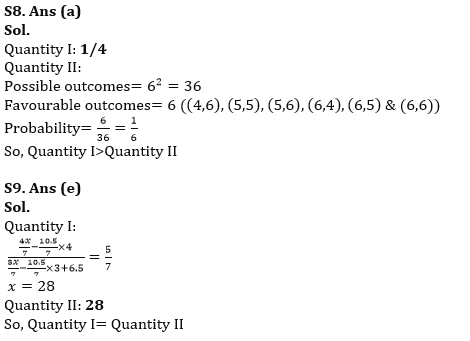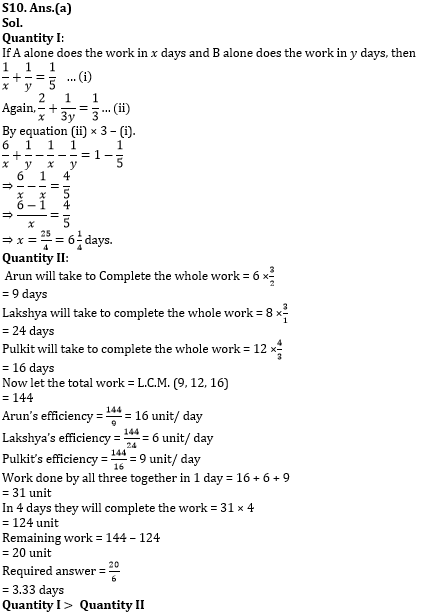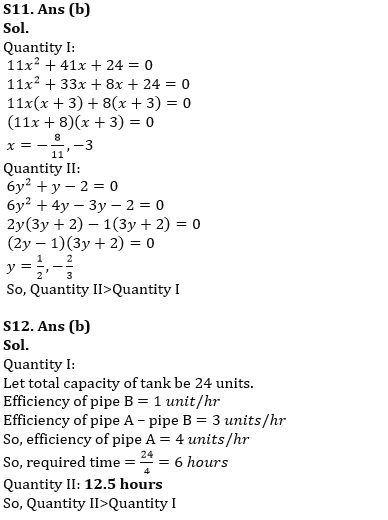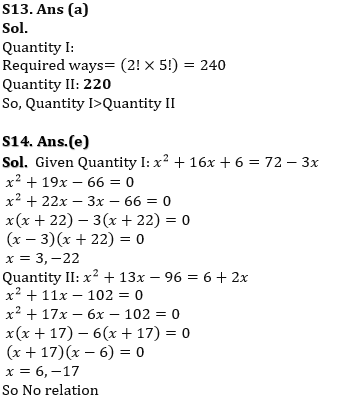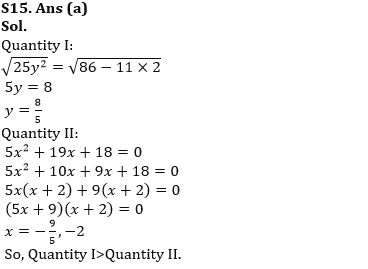Latest Banking jobs   »

# Quantitative Aptitude Quiz For RBI Grade B/ ECGC PO/ SIDBI Grade A Prelims 2022- 12th April

Directions (1-7): In each of the questions below two statements I and II are given. You have to decide whether the statement/statements is/are sufficient to answer the question.

Q1. Find the time taken by boat to cover 80 km downstream.
Statement I: The ratio of speed of boat in downstream to that in upstream is 9:7.
Statement II: The boat covers a distance of 24 km in 3 hours in still water.
(a)Only statement I itself is sufficient to answer the question.
(b) Only statement II itself is sufficient to answer the question.
(c)Either statement I or statement II is sufficient to answer the question.
(d)Both statement I and statement II are necessary to answer the question.
(e)Neither statement I nor statement II is sufficient to answer the question.

Q2. Find the rate of simple interest per annum?
Statement I: Anil invested Rs.6000 and received a total of Rs.7200 as an amount at the end of three years.
Statement II: Anil invested Rs. P for 2 years and received an amount of Rs.2800 as an interest at the end of two years.
(a)Only statement I itself is sufficient to answer the question.
(b)Only statement II itself is sufficient to answer the question.
(c)Either statement I or statement II is sufficient to answer the question.
(d)Both statement I and statement II are necessary to answer the question.
(e)Neither statement I nor statement II is sufficient to answer the question.

Q3. Find the length of the train A.
Statement I: Train A crosses another train B running in opposite direction in 27 seconds. The ratio of speeds of train A to train B is 6:5.
Statement II: Train A running at a speed of 90 kmph crosses a pole in 18 seconds.
(a)Only statement I itself is sufficient to answer the question.
(b) Only statement II itself is sufficient to answer the question.
(c)Either statement I or statement II is sufficient to answer the question.
(d)Both statement I and statement II are necessary to answer the question.
(e)Neither statement I nor statement II is sufficient to answer the question.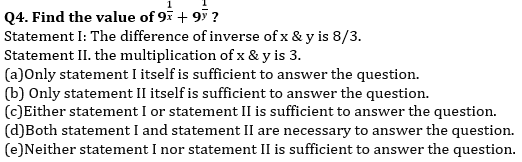Q5. Find the present age of Shivam? (in years)
Statement I: The ratio of present age of Shivam and Shubham is 5:3.
Statement II: The difference between the ages of Shivam and Shubham is 18 years.
(a)Only statement I itself is sufficient to answer the question.
(b) Only statement II itself is sufficient to answer the question.
(c)Either statement I or statement II is sufficient to answer the question.
(d)Both statement I and statement II are necessary to answer the question.
(e)Neither statement I nor statement II is sufficient to answer the question.

Q6. What is the profit/loss percentage on the article having cost price Rs.1800?
Statement I: The discount offered on the article is 25% of the selling price.
Statement II: The marked price of the same article is 30% above the cost price.
(a)Only statement I itself is sufficient to answer the question.
(b) Only statement II itself is sufficient to answer the question.
(c)Either statement I or statement II is sufficient to answer the question.
(d)Both statement I and statement II are necessary to answer the question.
(e)Neither statement I nor statement II is sufficient to answer the question.

Q7. Find the number of days taken by A and B together to complete the work together?
Statement I: The number of days taken by A to complete the work alone is 22.5 days and the ratio of efficiency of A to B is 4:5.
Statement II: The number of days taken by A, B & C together to complete the work is 6 days while C alone takes 18 days to complete that particular work.
(a)Only statement I itself is sufficient to answer the question.
(b) Only statement II itself is sufficient to answer the question.
(c)Either statement I or statement II is sufficient to answer the question.
(d)Both statement I and statement II are necessary to answer the question.
(e)Neither statement I nor statement II is sufficient to answer the question.

Directions (8-15): In each of the questions given below, read the given statements and compare the quantity I and quantity II to conclude the answer.

Q8. Quantity I: 1/4
Quantity II: Find the probability of getting a sum greater than 9 when two dices are thrown simultaneously?
(a)Quantity I>Quantity II
(b)Quantity II>Quantity I
(c)Quantity I≤ Quantity II
(d)Quantity I≥ Quantity II
(e)Quantity I= Quantity II or no relationship established.

Q9. Quantity I: A mixture of x liters of milk and water is in the ratio of 4:3. When 10.5 liters mixture is removed and 6.5 liters of water is added then ratio of water to milk changes to 7:5. Find the value of x.
Quantity II: 28
(a)Quantity I>Quantity II
(b)Quantity II>Quantity I
(c)Quantity I≤ Quantity II
(d)Quantity I≥ Quantity II
(e)Quantity I= Quantity II or no relationship established.

Q10. Quantity I: How many days A would require to complete the work, working alone.
If two workers A and B working together completed a job in 5 days and if A worked twice as efficiently as he actually did and B worked 1/3 as efficiently as he actually did, the work would have been completed in 3 days.
Quantity II: Arun completes 2/3 of a certain job in 6 days. Lakshya can complete 1/3 of the same job in 8 days and Pulkit can complete 3/4 of the work in 12 days. All of them work together for 4 days and then Arun and Pulkit quit. How long will it take for Lakshya to complete the remaining work alone?
(a) Quantity I > Quantity II
(b) Quantity I < Quantity II
(c) Quantity I ≥ Quantity II
(d) Quantity I ≤ Quantity II
(e) Quantity I = Quantity II

Q11. Quantity I. 11x²+41x+24=0
Quantity II. 6y²+y-2=0
(a)Quantity I>Quantity II
(b)Quantity II>Quantity I
(c)Quantity I≤ Quantity II
(d)Quantity I≥ Quantity II
(e)Quantity I= Quantity II or no relationship established.

Q12. Quantity I: Find the time taken by Pipe A to completely fill a tank, if Pipe A and another outlet pipe B together fill the same tank in 8 hours and Pipe B empties the tank in 24 hours? (in hours)
Quantity II: 12.5 hours
(a)Quantity I>Quantity II
(b)Quantity II>Quantity I
(c)Quantity I≤ Quantity II
(d)Quantity I≥ Quantity II
(e)Quantity I= Quantity II or no relationship established.

Q13. Quantity I: Find the number of ways in which “SILENT” can be arranged so that vowels always comes together?
Quantity II: 220
(a)Quantity I>Quantity II
(b)Quantity II>Quantity I
(c)Quantity I≤ Quantity II
(d)Quantity I≥ Quantity II
(e)Quantity I= Quantity II or no relationship established.

Q14. Quantity I: x²+16x+6=72-3x
Quantity II: x²+13x-96=6+2x
(a) Quantity I > Quantity II
(b) Quantity I < Quantity II
(c) Quantity I ≥ Quantity II
(d) Quantity I ≤ Quantity II
(e) Quantity I = Quantity II or No relation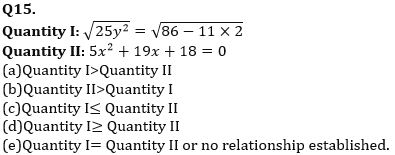Solutions